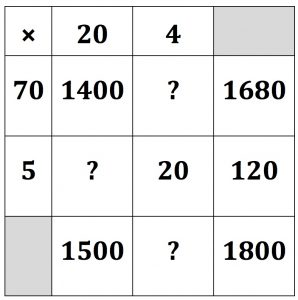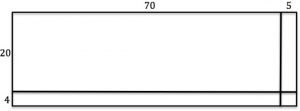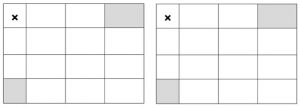Can you find numbers to replace the ? marks to solve this two by two multiplication puzzle? Can you explain how it works?

How does the diagram below connect with the multiplication?Use the two grids to do the two multiplications:

72 × 25 and 50 x 36

What do you notice? Why is this happening?